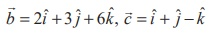Home | | Maths 12th Std | Equation of a plane passing through two given distinct points and is parallel to a non-zero vector

# Equation of a plane passing through two given distinct points and is parallel to a non-zero vector

(a) Parametric form of vector equation (b) Non-parametric form of vector equation (c) Cartesian form of equation

Equation of a plane passing through two given distinct points and is parallel to a non-zero vector

### (a) Parametric form of vector equation

The parametric form of vector equation of the plane passing through two given distinct points A and B with position vectorsand, and parallel to a non-zero vectoris### (b) Non-parametric form of vector equation

Equation (1) can be written equivalently in non-parametric vector form asWhere (-andare not parallel vectors.

### (c) Cartesian form of equationThis is the required Cartesian equation of the plane.

Example 6.43

Find the non-parametric form of vector equation, and Cartesian equation of the plane passing through the point (0,1, −5) and parallel to the straight linesSolution

We observe that the required plane is parallel to the vectorsand passing through the point (0,1, −5) with position vectorWe observe thatis not parallel to. Then the vector equation of the plane in non-parametric form is given by= 0.         …(1)If= xˆi + yˆj + zˆk is the position vector of an arbitrary point on the plane, then from the above equation, we get the Cartesian equation of the plane as -9x + 8 y - z = 13 or 9x - 8 y + z +13 = 0 .

### Example 6.44

Find the vector parametric, vector non-parametric and Cartesian form of the equation of the plane passing through the points (−1, 2, 0), (2, 2 −1) and parallel to the straight line### Solution

The required plane is parallel to the given line and so it is parallel to the vector= iˆ + ˆj − ˆk and he plane passes through the points= −ˆi + 2ˆj= 2ˆi + 2ˆj − ˆk .

vector equation of the plane in parametric form is, where s, t R which implies that= (-ˆi + 2ˆj ) + s (3ˆi - ˆk )+ t (ˆi + ˆj - ˆk ) , where s, t R.

vector equation of the plane in non-parametric form iswe have (- (-iˆ + 2ˆ j)) . (ˆi + 2ˆj + 3ˆk ) = 0. (ˆi + 2ˆ j + 3ˆk ) = 3

If= xˆi + yˆj + zˆk is the position vector of an arbitrary point on the plane, then from the above equation, we get the Cartesian equation of the plane as x + 2 y + 3z = 3 .

Study Material, Lecturing Notes, Assignment, Reference, Wiki description explanation, brief detail
12th Mathematics : UNIT 6 : Applications of Vector Algebra : Equation of a plane passing through two given distinct points and is parallel to a non-zero vector |